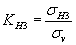Join one of our upcoming courses taking place around the world! Explore now

# Gravity Field Stress

Use the Gravity Field Stress option if you wish to specify an initial in-situ stress field which varies linearly with depth from a specified elevation.

• The Gravitational Field Stress option is only strictly applicable for horizontal excavations (i.e., Plunge = 0). If the excavation is more than a few degrees from horizontal or is vertical, then you should NOT use the Gravitational option, because the field stresses will not be calculated correctly. In this case, use the Constant Field Stress option.
• See the Field Stress topic for general information about using the Field Stress option in UnWedge.

The following parameters are required to define Gravitational Field Stress.

## Ground Surface Elevation

The Ground Surface Elevation is the y-coordinate of the ground surface and MUST correspond to the coordinates with which you have defined your Opening Section. For example:

• If the uppermost vertex of the Opening Section boundary has a y-coordinate = 50 meters, and you want to define a Ground Surface which is 10 meters above this point, then enter a Ground Surface Elevation = 60 meters.

Remember, the Ground Surface Elevation is the y-coordinate of the ground surface. It is NOT the depth of the excavation from the surface.

If a ground surface exists, the defined ground surface will be used and the Ground Surface Elevation input box will be disabled.

## Trend of Major Horizontal Stress

For the Gravitational Field Stress option, one of the principal stress directions is always the VERTICAL direction.

The orientation of the other two principal stress directions is then defined by specifying a Trend of Major Horizontal Stress.

Because UnWedge uses a Complete Plane Strain formulation, this means that the principal stress orientations do not have to be aligned with the excavation axis. Although the excavation model is 2-dimensional, the defined Field Stress (and the stress analysis results) can be 3-dimensional.

## Major and Minor Horizontal Stress Ratios

The magnitudes of the Major and Minor Horizontal principal stresses are specified as ratios of the Vertical Stress. The Vertical Stress is determined by the depth of a point from the Ground Surface Elevation, and the Unit Weight of the rock, as follows:The Major Horizontal Stress Ratio is given by:The Minor Horizontal Stress Ratio is given by:These equations determine the magnitude of the in-situ principal stresses at any depth from the surface.

The Vertical Stress is not necessarily the overall Major Principal Stress (e.g. if the Major Horizontal Stress ratio was greater than 1, then the Vertical Stress could be the Intermediate or Minor overall principal stress).